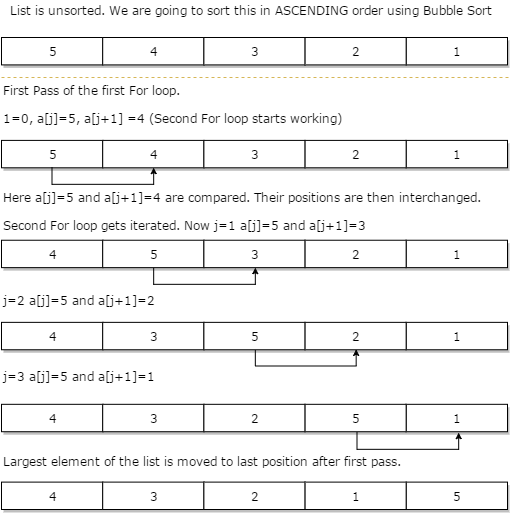## Data Structure Tutorial Index

Bubble Sort Algorithm is used to arrange N elements in ascending order, and for that, you have to begin with 0th element and compare it with the first element. If the 0th element is found greater than the 1st element, then the swapping operation will be performed, i.e., the two values will get interchanged. In this way, all the elements of the array get compared.

Below given figure shows how Bubble Sort works:### Algorithm for Bubble Sort

1. algorithm Bubble_Sort(list)
2. Pre: list != fi
3. Post: list is sorted in ascending order for all values
4. for i <- 0 to list:Count - 1
5. for j <- 0 to list:Count - 1
6. if list[i] < list[j]
7. Swap(list[i]; list[j])
8. end if
9. end for
10. end for
11. return list
12. end Bubble_Sort